### SOLUTIONS TO INTEGRATION BY PARTS

SOLUTION 16 : Integrate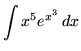. Note that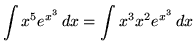.

Now let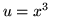andso that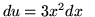and.

Therefore,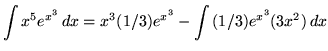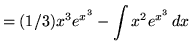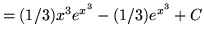.

SOLUTION 17 : Integrate. Note that.

Letandso thatand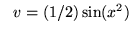.

Therefore,.

SOLUTION 18 : Integrate. Note that.

Letand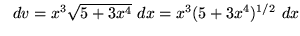so thatand.

Therefore,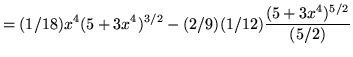+ C+ C .

SOLUTION 19 : Integrate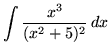. Note that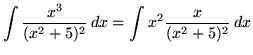.

Letandso thatand.

Therefore,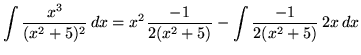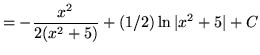.

SOLUTION 20 : Integrate. Note that.

Let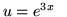and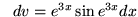so that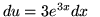and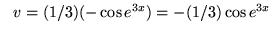.

Therefore,.

SOLUTION 21 : Integrate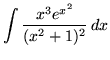. Note that.

Let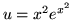and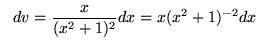so thatand.

Therefore,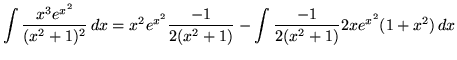.

SOLUTION 22 : Integrate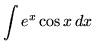. Letandso thatand.

Therefore,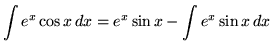.

Use integration by parts again. letandso thatand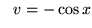.

Hence,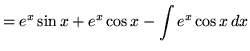.

To both sides of this "equation" add, getting.

Thus,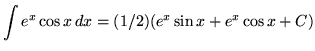(Combine constant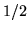withsinceis an arbitrary constant.).

SOLUTION 23 : Integrate. Use integration by parts. Let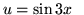and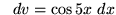so that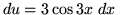and.

Therefore,.

Use integration by parts again. let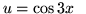andso thatand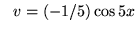.

Hence,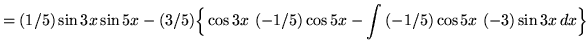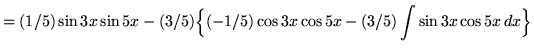.

From both sides of this "equation" subtract, getting.

Thus,(Combine constantwithsinceis an arbitrary constant.)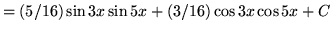.